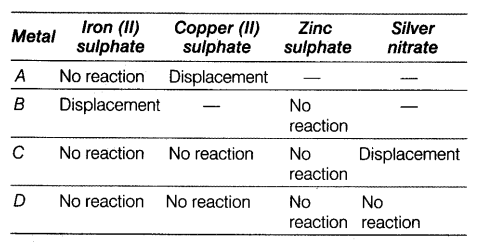# Four metals A, B, C and D are added to the following solutions one by one

Four metals A, B, C and D are added to the following solutions one by one. The observations made are tabulated below:Answer the following questions based on the above information:
(i) Which is the most active metal and why?
(ii) What would be observed if B is added to a solution of copper sulphate and why?
(iii) Arrange the metals A,B,C and D in order of increasing reactivity.
(iv) Container of which metal can be used to store both zinc sulphate solution and silver nitrate solution?
(v) Which of the above solutions can be easily stored in a container made up of any of these metals?

(i) B is the most active metal as it displaces iron from its salt solution.
(ii) B will displace Cu from CuSO4 solution because B is more reactive than copper.
(iii) D < C <A < B
(iv) Container of metal D can be used to store both \$ZnSO_{4}\$ and \$AgNO_{3}\$ solutions, because it cannot displace Zn and Ag from their salt solutions.
(v) \$ZnSO_{4}\$ can be stored in a container made up of any of these metals.

1 Like